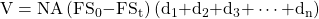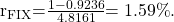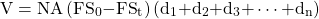Essential Concept 78: Interest Rate Swaps | IFT World
101 Concepts for the Level I Exam

# Essential Concept 78: Interest Rate SwapsA swap is an over-the-counter contract between two parties to exchange a series of cash flows based on some pre-determined formula. In a plain vanilla interest rate swap one party pays fixed rate, whereas the other party pays a floating rate.

Swap fixed rate = (1 – final discount factor) / (sum of all discount factors)

Value of a fixed rate swap at Time t = Sum of the present value of the difference in fixed swap rates x Stated notional amountExample: Suppose two years ago we entered a €100,000,000 seven-year receive-fixed Libor-based interest rate swap with annual resets using 30/360 day count. The fixed rate in the swap contract at initiation was 3%. Using the present value factors in the below given table, what is the value for the party receiving the fixed rate?

 Maturity (years) Present Value Factors 1 0.9906 2 0.9789 3 0.9674 4 0.9556 5 0.9236

Solution: We first compute the new swap fixed rate. The sum of the present values is 4.8161. Therefore, the fixed swap rate is:V = (0.03 – 0.0159) 4.8161 × 100,000,000 = €6,790,701

Thus, the swap value is €6,790,701.